Function Repository Resource:

# SimplifyRepeatedSubexpressions

Replace repeated subexpressions in an expression with new symbols

Contributed by: Jon McLoone
 ResourceFunction["SimplifyRepeatedSubexpressions"][expr] returns a list of the expr with repeated subexpressions removed, along with the replacement rules used to rewrite them in simpler form.

## Details and Options

ResourceFunction["SimplifyRepeatedSubexpressions"] supports the following options:
 "MinLeafCount" 2 minimum size of the subexpressions to be extracted "VariableNames" Unique[]& method to generate the names of the subexpressions

## Examples

### Basic Examples (2)

Find the repeated term in the expression (a+b+c)3+(a+b+c)2:

 In:=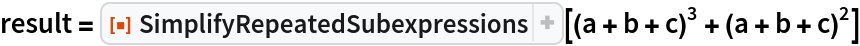Out=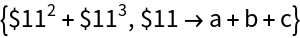Reconstruct the original expression from the decomposed form:

 In:=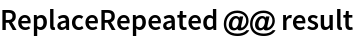Out=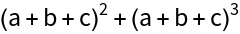### Options (6)

Finding many small common subexpressions may not be helpful:

 In:=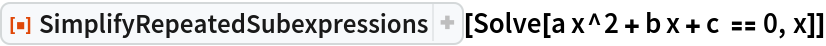Out=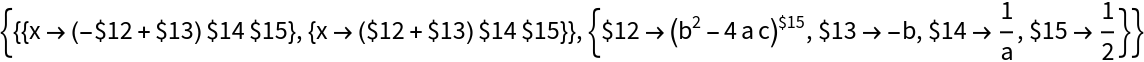Find only the largest with "MinLeafCount":

 In:=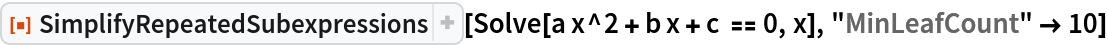Out=You can control the prefix used for subexpressions by providing a string:

 In:=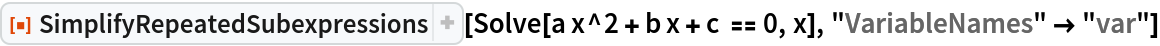Out=You can also control the names used for subexpressions by providing a list of variable names:

 In:=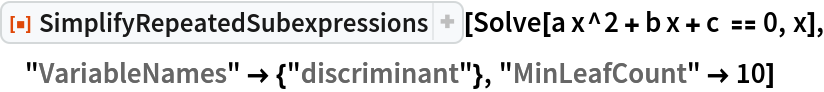Out=Or by providing a generating function:

 In:=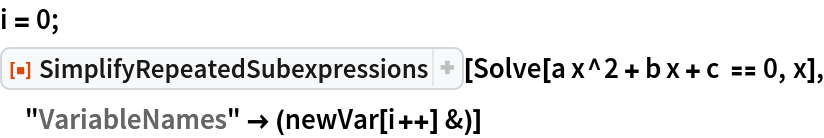Out=The "VariableNames" option takes the subexpression as an argument:

 In:=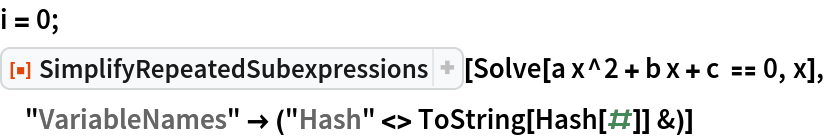Out=### Applications (1)

Remove the discriminant from the quadratic solution equations:

 In:=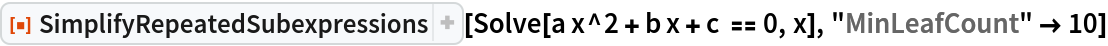Out=### Properties and Relations (1)

Reconstruct the original expression from the decomposed form by applying ReplaceRepeated:

 In:=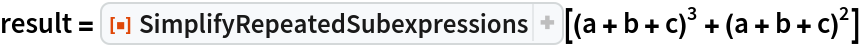Out=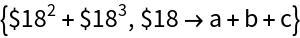In:=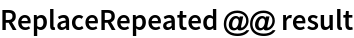Out=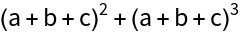### Possible Issues (2)

The FullForm of subexpressions must match; in this case, no match is found:

 In:=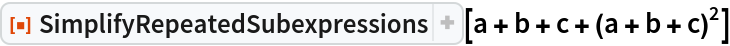Out=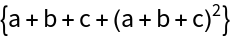This is because the outermost Plus has four arguments and not just the a, b and c:

 In:=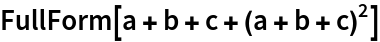Out=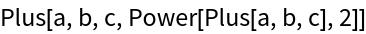If the input expression contains elements with HoldAll, HoldRest or HoldFirst, the contents will evaluate during the search:

 In:=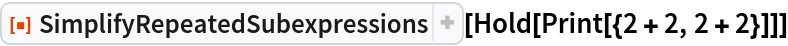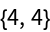Out=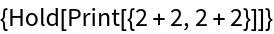### Neat Examples (3)

Simplify the third-degree polynomial solution:

 In:=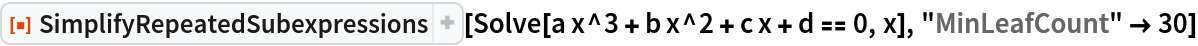Out=And the fourth-degree polynomial solution:

 In:=Out=Construct meaningful variable names based on their content:

 In:=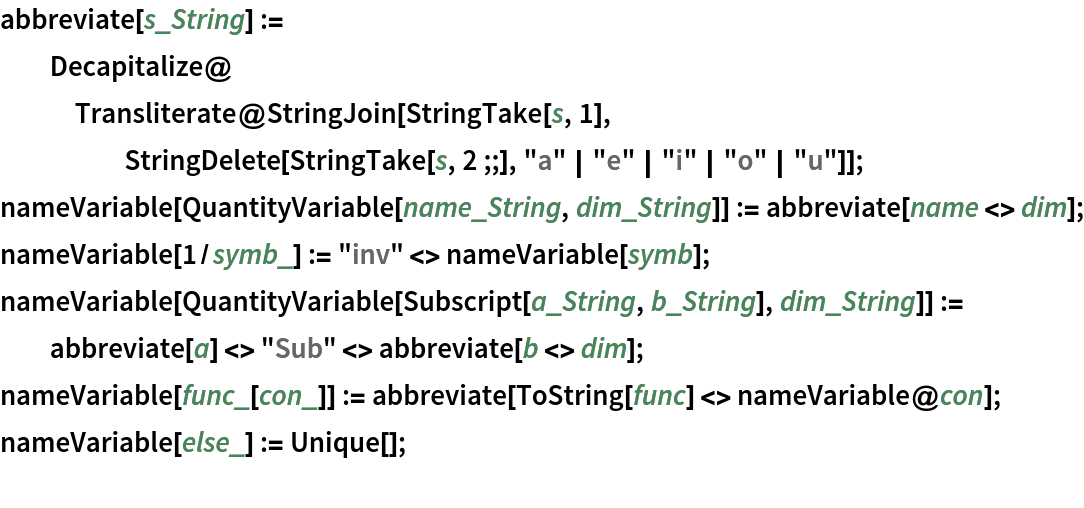In:=Out=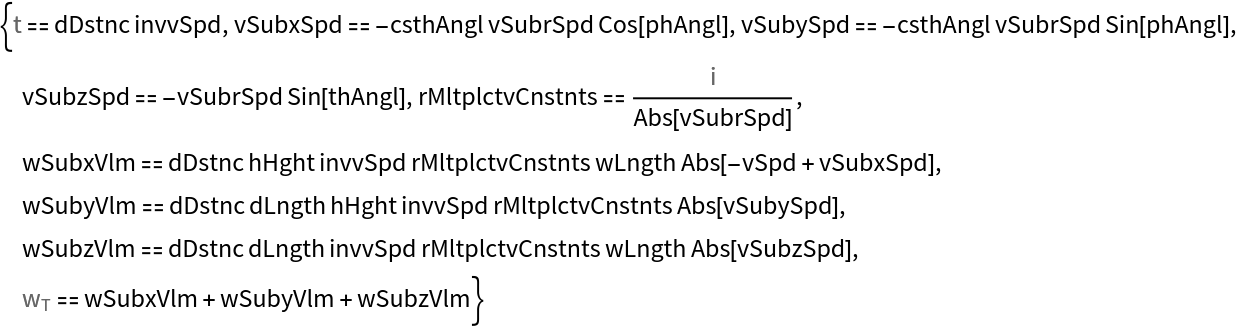Jon McLoone

## Requirements

Wolfram Language 11.3 (March 2018) or above

## Version History

• 1.1.0 – 29 November 2021